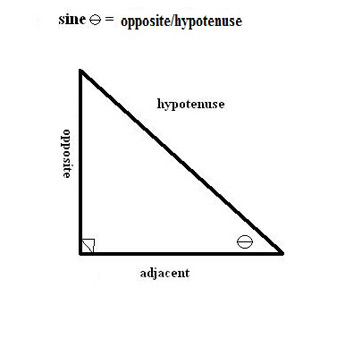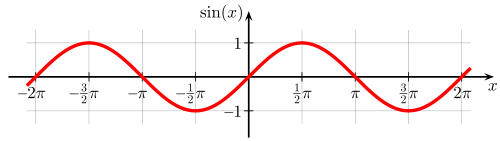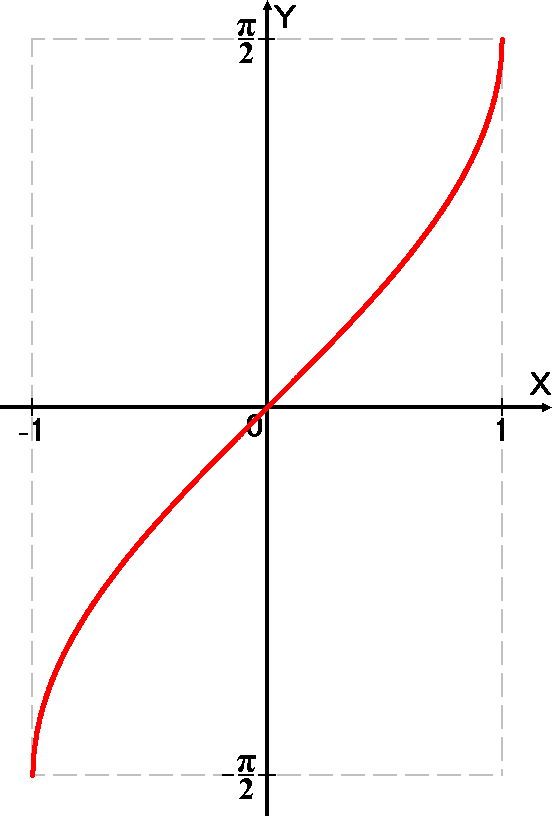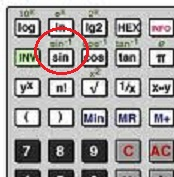# Arcsine: Definition & Overview

Instructor: Jennifer Beddoe

Jennifer has an MS in Chemistry and a BS in Biological Sciences.

The arcsine is the inverse operation of the sine function. This lesson will give a definition of the arcsine and provide examples of how it can be used to find the measure of an angle. A quiz following the lesson will help cement what you've learned.

## Inverse Operations

In mathematics, most operations have an inverse operation. The inverse operation is the opposite of the original operation. So, addition is the opposite of subtraction and division is the inverse of multiplication. Squared is the inverse of the square root.

Trigonometric functions are no different. They all have inverse operations.

• The inverse of sine is arcsine.
• The inverse of cosine is arccosine.
• The inverse of tangent is arctangent.
• The inverse of secant is arcsecant.
• The inverse of cosecant is arccosecant.
• The inverse of cotangent is arccotangent.

Inverse trigonometric functions have practical applications in physics, engineering, and navigation.

## SineThe sine (sin) of an angle in a right triangle is a trigonometric function that can be defined as the ratio of the side opposite the angle to the hypotenuse.The sine function can be used to solve problems involving a right triangle. For example:

A railroad track is inclined at 1°. If the train travels 3000 meters up the hill, what is the vertical height that the train will have traveled?

sin'x' = opposite/hypotenuse

sin1° = opposite/3000 m

opposite = (sine1°)(3000 m)

opposite = 52.36 m

So, the train rises 52.36 meters.

## Arcsine

The arcsine is the inverse function of the sine function. It is most useful when trying to find the angle measure when two sides of a triangle are known.To solve problems using the arcsine, you need a scientific calculator. It should have a button, usually above the sin button.The arcsine will allow you to find the measure of an angle when you know the ratio of the side opposite the angle to the hypotenuse.

## Examples

1.) Find the angle measure Θ in the following triangle.

sin'Θ' = 7/13

sin'Θ' = 0.538

x = 57.4°

2.) Let's look at the railroad example from above. If you knew that the train rose 3 vertical miles on a track that was 5 miles long, you could determine the angle of incline of the track using the arcsine.

sin'x' = 3 miles/5 miles (opposite/hypotenuse)

sin 'x' = 0.6

To unlock this lesson you must be a Study.com Member.
Create your account

### Register to view this lesson

Are you a student or a teacher?

### Unlock Your Education

#### See for yourself why 30 million people use Study.com

##### Become a Study.com member and start learning now.
Back
What teachers are saying about Study.com
Create an account to start this course today
Used by over 30 million students worldwide